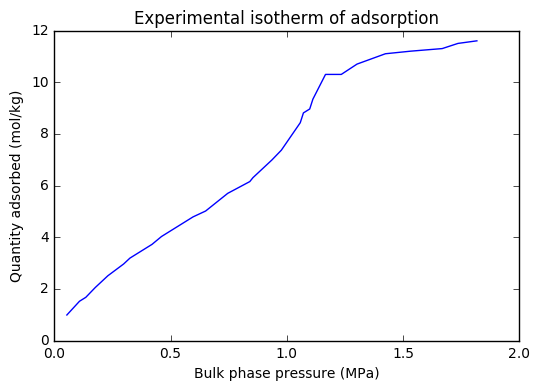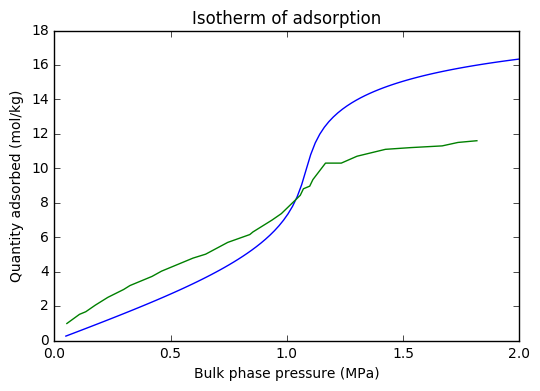# Calculation of adsorption for pure fluids by Equation of State¶

Mariana Amorim de Almeida $^a$ (January 2017)

$a$) Escola de Química, Universidade Federal do Rio de Janeiro, Rio de Janeiro, Brasil

### Thermodynamic equilibrium condition for confined fluids¶

For a closed system, the first and second laws of Thermodynamics establishes that:

$dU-TdS+PdV \leq 0$

in which U is the internal energy, S is entropy, P is pressure of the system and V is volume of the sistem. However, for confined fluids it is more convenient to work with specified temperature, confining volume and chemical potential. For this specifications, the system does not have a constant mass. Thus, to consider the mass variation of this system, the previous inequality must be written as follows:

$dU_a-T_adS_a+P_adV_a-\mu_adn_a\leq0$

in which $n_a$ is the confined fluid mole number and the index a identifies the adsorbed phase. So, rearranging the inequality for $V_a$ constant:

$d(U_a-T_aS_a-\mu_an_a)\leq0$

From the definition of Gibbs energy:

$G=\mu n=U+PV-TS$

Substituting the definition of the Gibbs energy in the inequality:

$d(-P_aV_a)\leq0$

As the confining volume is constant, the thermodynamic equilibrium condition imposes that:

$dP_a\geq0$

So, for specified temperature, confining volume and the chemical potential, the termodynamic equilibrium condition for the confined fluid is the maximization of its pressure.

### The adsorption problem¶

The adsorption phenomenon can be understood as the accumulation of substances in an interface. In this work, we are interested in systems in which a fluid accumulates in a porous solid. The adsorption problem is then characterized by the phase equilibrium between the bulk phase and the adsorbed phase in the solid, and it is possible to write the following equilibrium equation, where v is the volumetric phase index and a is the adsorbed phase index.

$\mu_i^v=\mu_i^a$

In general, what is desired to obtain from this equation is the density of the adsorbed phase with which it is possible to calculate the total amount of fluid adsorbed in the solid. In this work, the steps that lead to obtaining the density of the adsorbed phase will be developed when a equation of state is used to describe both phases in equilibrium.

### Equation of state for confined fluids¶

In the adsorption systems, the adsorbed phase is under different conditions from the bulk phase. This is because, within the pores, the interactions between the fluid molecules and the walls of the solid are relevant, changing the thermodynamic and structural properties of the fluid. In order to study the properties of fluids under these conditions, Travalloni et al. (2010) extended to confined fluid modeling some cubic equations of state, widely used in engineering, using the Generalized van der Waals Theory. The models obtained by the authors are able, through empirical expressions, to model the effects of confinement on the properties of the fluids being dependent on variables of the fluid/solid system. In this work, the model obtained by the authors from the Peng-Robinson equation of state will be used. It is worth mention that all the equations developed in the referred work retake the original equation on which they were based, in their volumar limit. In this way, the same equation can be used to obtain the properties of the volumar and adsorbed phases.

### Calculation of adsorption for pure fluids by Equation of State¶

One of the necessary conditions for the phases of the adsorption system to be in thermodynamic equilibrium is determined by the equality of chemical potentials that reappears below with its explicit independent variables.

$\mu_v (T,P_v;a,b)=\mu_a(T,\rho_a,r_p;a,b,\epsilon_p,\delta_p)$

In this equation, $T$ is the system temperature, $P_v$ is the bulk phase pressure, $a$ e $b$ are the parameters of the Peng-Robinson equation of state, $\rho_a$ is the density of the adsorbed phase, $r_p$ is the solid pore radius considering its cylindrical geometries, $\epsilon _p$ e $\delta_p$ are the interaction parameters between the fluid molecules and the walls of the solid considering square well potential. To perform the adsorption calculations, it is necessary to specify $T$, $P_v$, $r_p$, the total volume of pores in the adsorbent ($V_p$) and the parameters $a$, $b$ , $\epsilon _p$ e $\delta_p$. The chemical potential of the system is then defined according to that referring to the volumar phase. The model used in this work can provide multiple real roots for a given set of specifications. Only those that satisfy the condition of mechanical stability, represented below, have physical meaning. Moreover, as demonstrated by Travalloni et al. (2010), damong the solutions found, the one that results in the highest pressure value of the adsorbed phase is the most stable.

$\frac{dP_a}{d\rho_a}>0$

In addition, the Topliss method was modified to explicit equations in chemical potential and it will be used here to obtain the real and mechanically stable roots that solve the adsorption problem. Note that the Topliss Method should only be used in the case of systems with at most three real roots, thus, the conditions studied here were limited to this restriction. Considering that the adsorbed amount corresponding to the total amount of adsorbed fluid on the solid, the number of adsorbed moles ($n_a$) can be calculated according to the equation below:

$n_a=V_p\rho_a$

# Tools¶

In :
import numpy as np
from scipy import optimize
import matplotlib.pyplot as plt
%matplotlib inline


# Fluid/solid system data¶

In :
#constants
R = 8.314 #Constant of ideal gases
Nav = 6.022*(10**23) #Avogadro's number
T = 264.75 #temperature
Pi = 3.14159265359
#fluid
#Tc = 190.6 #critical temperature methane
#Pc = 45.99*10**5 #critical pressure methane
#w = 0.012 #accent factor methane
Tc = 305.3 #critical temperature ethane
Pc = 48.72*10**5 #critical pressure ethane
w = 0.100 #accent factor ethane
a = 0.45724*R**2*Tc**2/Pc #parameter of EOS Peng-Robinson
b = 0.0778*R*Tc/Pc #parameter of EOS Peng-Robinson
sigma = (1.15798*b/Nav)**(1/3) #characteristic length of molecules
alfa = (1+(0.37464+1.54226*w-0.26992*w**2)*(1-(T/Tc)**0.5))**2
#sólido: MCM41
Rcap = 2.04*10**(-9) #mean pore radius of the solid
h = 7.9616384734967691*10**10 #sum of all pore heights considering cylindrical pores
#deltap = 0.38144*sigma #parameter of the square well potential for methane
#epspk = 702.24 #parameter of the square well potential normalized by k for methane
deltap = 0.78113*sigma #parameter of the square well potential for ethane
epspk = 797.82 #parameter of the square well potential normalized by k for ethane


# Empirical expressions for confined fluids¶

In :
#expressions
romax = (1.1579783713281564+4.427705489078004/np.exp(4.013769497416034*Rcap/sigma)-0.653722697044913/np.exp(0.6208605306104413*Rcap/sigma))/(Nav*sigma**3) #molar density in the compaction condition
Fpr = ((Rcap-sigma/2)**2-(Rcap-sigma/2-deltap)**2)/(Rcap-sigma/2)**2 #fraction of molecules that occupy the pore region under attractive influence of the wall in the random distribution
teta = Rcap/(sigma/2+deltap) #modulator of the effect of the fluid density on the distribution of the molecules inside the pore
ncg = 1-2*sigma/(5*Rcap) #modulator of the confinement effect on the reduction of the coordination number of the fluid


# Discretization of Pressures¶

In :
#pressures for methane
#P_i = 50000
#P_f = 3100000
#nP = 100
#step = (P_f-P_i)/nP
#P = np.arange(P_i,P_f+step,step)

#pressures for ethane
P_i = 50000
P_f = 2000000
nP = 100
step = (P_f-P_i)/nP
P = np.arange(P_i,P_f+step,step)


# Functions¶

• Bulk phase volume function
In :
#calculation of v bulk
def function_vb(T,P):

c3 = P #coefficient for v^3
c2 = 3*P*b-R*T #coefficient v^2
c1 = -2*b*R*T+alfa*a-3*P*(b**2)  #coefficient v^1
c0 = R*T*(b**2)-alfa*a*b+P*(b**3)  #coefficient v^0

RES = 1
TOL = 1e-9
MAX = 1000
i = 0
vb = R*T/P
vb2 = 0
vb3 = 0
while(RES>TOL and i<MAX):
vb = vb-(c3*vb**3+c2*vb**2+c1*vb+c0)/(3*c3*vb**2+2*c2*vb+c1)
RES = c3*vb**3+c2*vb**2+c1*vb+c0
i = i+1
if vb < b:
vb = 0

# calc r2 and r3
c4 = c3
c5 = c2+c4*vb
c6 = c1+c5*vb

delta = (c5**2)-(4*c4*c6)

if delta >= 0:
vb2 = (-c5+np.sqrt(delta))/(2*c4)
if vb2 < b:
vb2 = 0
vb3 = (-c5-np.sqrt(delta))/(2*c4)
if vb3 < b:
vb3 = 0
else:
vb2 = 0
vb3 = 0

vb,vb2,vb3 = np.sort([vb,vb2,vb3])

return [vb,vb2,vb3]

• Function of calculation of chemical potential of the volumar phase
In :
def function_mib(T,v_bulk):
vb = v_bulk
mib = (-(1/4))*R*T*(4*(b/(b-vb)+np.log(-b+vb))+(a*alfa*((4*vb)/(-b**2+2*b*vb+vb**2)+(np.sqrt(2)*(-(np.log(b-np.sqrt(2)*b+vb))+np.log(b+np.sqrt(2)*b+vb)))/b))/(R*T))
return mib

• Function of calculating the adsorbed phase pressure
In :
def function_pa(T,ro_ads):
pa = R*roa*T*(romax*(1/(-roa+romax)+(a*alfa*ncg*roa*romax)/(R*roa**2*T-2*R*roa*romax*T-R*romax**2*T))+((-1+Fpr)*roa*(1-roa/romax)**teta*(np.exp(epspk/T)*(epspk-T)+T)*teta)/(np.exp(epspk/T)*((roa-romax)*T)))
return pa

• Function of calculation of the chemical potential of the adsorbed phase
In :
def function_mia(T,ro_ads):
mia = (-(1/4))*R*T*(4*np.log(1/roa-1/romax)+(1/T)*((1/(roa-romax))*(4*(((roa-romax)*((-a)*alfa*ncg*roa*romax**2+epspk*Fpr*R*(roa**2-2*roa*romax-romax**2)))/(R*(roa**2-2*roa*romax-romax**2))+roa*T-((-1+Fpr)*(1-roa/romax)**teta*(np.exp(epspk/T)*(epspk-T)+T)*(roa-romax+roa*teta))/np.exp(epspk/T)))+(np.sqrt(2)*a*alfa*ncg*romax*np.log((roa+np.sqrt(2)*roa+romax)/(roa-np.sqrt(2)*roa+romax)))/R))
return mia

• Function of calculating the first derivative of the chemical potential of the adsorbed phase in relation to the adsorbed density
In :
def function_dmiadroa(T,ro_ads):

• Objective function for calculating adsorbed density
In :
def function_Fobj(T,ro_ads):
Fobj = function_mia - mi_ads
return Fobj


# Modified Topliss’s method¶

The number of real solutions that the equilibrium condition will provide depends on the appearance of the $\mu_a$ vs. $\rho_a$ curve. In this work, the aspects of the mentioned curve that provide up to three real solutions will be analyzed. The $\alpha$ aspect will always provide a single solution to the equilibrium condition, regardless of the $\mu$ specification of the problem. However, the $\beta$ aspect, may provide one or three solutions according to the $\mu$ specification of the problem. To determine the appearance of the $\mu_a$ vs. $\rho_a$ studied is necessary to calculate the point at which the $\mu_a$ derivative to $\rho_a$ is minimal. If this point, called point A is positive, the curve looks $\alpha$ and the problem has only one solution. If point A is negative, the number of solutions is not determined yet. The next step of the method is the calculation of the B and C points, both occur when the derivative of $\mu_a$ in relation to $\rho_a$ is null, however, B has less $\rho_a$ than C. It is then necessary to compare the $\mu_a$ specification of the problem to the $\mu_a$ calculated at points B and C. If $\mu_a$ is less than the potential at point B and greater than the potential at point C, the problem has only one solution. If $\mu_a$ is among the potentials calculated for points B and C the problem presents three solutions of which only those that respect the stability criteria will be analyzed..

In :
def function_roa(mi_ads):
#Determination of point A
PtoA = sol.x
if PtoA >  0:
roa_aux = optimize.bisect(lambda ro_ads: function_mia(T,ro_ads) - mi_ads, 0.001*romax, 0.9*romax, xtol=2e-12, rtol=8.8817841970012523e-16, maxiter=100)

else:
#Determination of points B and C

PtoB = function_mia(T,roa_B)
PtoC = function_mia(T,roa_C)
if PtoB > mi_ads or PtoC < mi_ads:
ro_aux = optimize.bisect(lambda ro_ads: function_Fobj(T,ro_ads), 0.001*romax, 0.9*romax, xtol=2e-12, rtol=8.8817841970012523e-16, maxiter=100)

else:
ro_aux_menor = optimize.bisect(lambda ro_ads: function_mia(T,ro_ads) - mi_ads, 0.001*romax, roa_B, xtol=2e-12, rtol=8.8817841970012523e-16, maxiter=100)
ro_aux_menor = optimize.bisect(lambda ro_ads: function_mia(T,ro_ads) - mi_ads, roa_C, 0.9*romax, xtol=2e-12, rtol=8.8817841970012523e-16, maxiter=100)

pa1 = function_pa(T,ro_aux_menor)
pa2 = function_pa(T,ro_aux_maior)
#Stability criterion
if pa1 > pa2:
roa_aux = ro_aux_menor
else:
roa_aux = ro_aux_maior
return roa_aux


# Calculation of adsorbed amount¶

In :
#volume calculation for the entire isotherm pressure grid
v_bulk = np.zeros()
for i in range (0,nP+1):
v_bulk = function_vb(T,P[i])
nroots = np.count_nonzero(v_bulk)
if (nroots == 1):
mi_bulk = function_mib(T,np.max(v_bulk))
elif (nroots == 3):
mi_bulk = np.min([function_mib(T,v_bulk),function_mib(T,v_bulk),function_mib(T,v_bulk)])

In :
plt.title('Experimental isotherm of adsorption')
plt.xlabel('Bulk phase pressure (MPa)')
#methane
#P_exp = np.sort([53640,67050,93870,120690,201150,375480,670500,965520,1059400,1287400,1609200,1703100,2078500,2212600,2614900,2816100,3097700])
#ethane
P_exp = np.sort([1817800,1736400,1668600,1532900,1424400,1302300,1234500,1166700,1112400,1098800,1071700,1058100,976740,936050,854650,841090,746120,651160,596900,461240,420540,352710,325580,298450,230620,176360,135660,108530,54264])
qads = np.sort([0.0115,0.0116,0.0113,0.0112,0.0111,0.0107, 0.0103,0.0103,9.3418*10**-3,8.9620*10**-3,8.8101*10**-3,8.4304*10**-3,7.3671*10**-3,6.9873*10**-3,6.3038*10**-3,6.1519*10**-3,5.6962*10**-3,5.0127*10**-3,4.7848*10**-3,4.0253*10**-3,3.7215*10**-3,3.3418*10**-3,3.1899*10**-3,2.9620*10**-3,2.5063*10**-3,2.0506*10**-3,1.6709*10**-3,1.5190*10**-3,9.8734*10**-4])
plt.show()# Isotherm¶

In :
plt.title('Isotherm of adsorption')